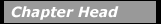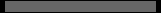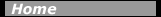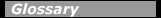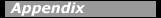## Units of Radioactivity and DoseThe original unit for measuring the amount of radioactivity was the curie (Ci)–first defined to correspond to one gram of radium-226 and more recently defined as:

1 curie = 3.7x1010 radioactive decays per second [exactly].

In the International System of Units (SI) the curie has been replaced by the becquerel (Bq), where

1 becquerel = 1 radioactive decay per second = 2.703x10-11 Ci.

The magnitude of radiation exposures is specified in terms of the radiation dose. There are two important categories of dose:

1. The absorbed dose, sometimes also known as the physical dose, defined by the amount of energy deposited in a unit mass in human tissue or other media. The original unit is the rad [100 erg/g]; it is now being widely replaced by the SI unit, the gray (Gy) [1 J/kg], where 1 gray = 100 rad.
• The biological dose, sometimes also known as the dose equivalent, expressed in units of rem or, in the SI system, sievert (Sv). This dose reflects the fact that the biological damage caused by a particle depends not only on the total energy deposited but also on the rate of energy loss per unit distance traversed by the particle (or "linear energy transfer"). For example, alpha particles do much more damage per unit energy deposited than do electrons. This effect can be represented, in rough overall terms, by a quality factor, Q. Over a wide range of incident energies, Q is taken to be 1.0 for electrons (and for x-rays and gamma rays, both of which produce electrons) and 20 for alpha particles. For neutrons, the adopted quality factor varies from 5 to 20, depending on neutron energy.
• The biological impact is specified by the dose equivalent H, which is the product of the absorbed dose D and the quality factor Q: H = Q D.

The unit for the dose equivalent is the rem if the absorbed dose is in rads and the sievert (Sv) if the absorbed dose is in grays. Thus, 1 Sv = 100 rem. As discussed below, 1 rem is roughly the average dose received in 3 years of exposure to natural radiation. 1 Sv is at the bottom of the range of doses that, if received over a short period of time, are likely to cause noticeable symptoms of radiation sickness.

The dose equivalent is still not the whole story. If only part of the body is irradiated, the dose must be discounted with an appropriate weighting factor if it is to reflect overall risk. The discounted dose is termed the effective dose equivalent or just the effective dose, expressed in rems or sieverts.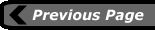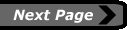last updated: August 9, 2000 webmaster